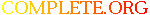Complete.Org: Mailing Lists: Archives: freeciv-ai: May 2002: [freeciv-ai] Amortize# [freeciv-ai] Amortize

[Top] [All Lists]

 To: freeciv-ai@xxxxxxxxxxx Subject: [freeciv-ai] Amortize From: Raimar Falke Date: Wed, 22 May 2002 18:54:39 +0200 Reply-to: rf13@xxxxxxxxxxxxxxxxxxxxxx

```[ since integral is longer than area and I have no idea how to draw an
integral symbol here I use the term area ]

Some definitions:
- delay: number of turns that you have to wait till the action will
produce something.
- surplus(turn) or (s(t) for short):
- t>=0
- t is relative shifted by current_turn+delay. So s(0) is really
s(current_turn+delay).
- may be < 0
- constant surplus: s(t)=const for all t (simple terrain improvements
have a constant surplus)
- non-constant surplus: example is the founding of a new city. The
surplus depends on the city size and this size isn't fixed.
- discrete surplus: s(t) is only defined for integer values of t
- decay function: d(t)
- d(0)=1
- d(t)=0 if t->inf
- d(t) is monotonic
- example d(t)=0.5^t

Problem 1: we want to decide weither an action A1 which has a delay of
delay_a1 and a surplus_a1 should be taken or action A2.

First solution: draw up the s(t) and calculate the area under the
curve.

Problem 1': the area is in most cases infinite.

Problem 2: we want to punish long delays.

Solution 2.1: we multiply the surplus with a decay function. So larger
delay give smaller overall surplus.

Solution 2.2: _if_ we had finite areas we don't need to care about the
delay since it will just reduce the area.

Solution 2.1 is the one used in current code. As d(t) (23/24)^t is
used. This means a decay rate of 1/24 ~= 4.16%. So this decay function
has a halflife of ln(2)/(1/24)=ln(2)*24 ~= 16.635 turns.

Note that it is also possible to calculate area_under(s(t)*d(t)) and
not just s(t)*d(t) (for s(t)=const).
S=s(t)
area_under(s(t)*d(t))=area_under(S*(23/24)^t)
=S*area_under((23/24)^t)
=S*(23/24)^t/ln(23/24)

So this is the same as the code calculates now expect a constant
factor. This factor however can be removed because it has no influence
on the comparison of the values.

Note that the current code can only handle constant surplus. If there
is a non-constant surplus you can either use a symbolic integration
(hard to very hard) or a numberic integration (also not easy, note
that the time horizon is unlimited).

Back to problem 1'. This can be solved if we limit our time horizon
from infinite to some finite value. This also allows us to easy
calculate the area. And it takes care of the delay problem. However it
introduces a new one: which time horizon to choose.

So both solutions have one parameter (the decay function/rate and the
time to consider) which can't intuitively be guessed.

Raimar

--
email: rf13@xxxxxxxxxxxxxxxxx
"Python is executable pseudocode. Perl is executable line noise"
-- Bruce Eckel

```